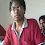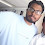# Pharma Engineering

For Engineer By Engineer

• ## Monday 21 March 2016

Hello everyone, Based on requests received asking how to determine the theoretical distillation time cycle in a batch reactor, and today i'm gonna demonstrate you a simple way to determine outta many critical ways to do that,

Today this determination of Time required to carry out distillation is Based upon the Reactor Heat transfer area.

How to find heat transfer area before proceeding to determination of distillation time

So, initially we need some of the data regarding

* The reactor mechanical design which includes some parameters like shell thickness, inner dia, length of reactor,

* The batch input details like how much amount of volume we need to handle, how much amount we need to distill out,

* The properties of the material we need to handle like density, specific heat, composition etc, and on the other end we need some properties like thermal conductivity of materials which out heat transfer area is composed off.

Properties of Materials:
Usually for make of Heat Transfer surface we will be usually going for SS316 Grade and for other purposes like for preparing the limpet jacketed coil and the insulation coating, we will be using SS304, and occasionally we will use GLR for Ph sensitive distillation, so below are the thermal conductivities of these materials

Material                Thermal Conductivity,K [W/m.K]
SS304                         10-13
SS316                         13-17
MSGLR                     1.2-1.5

Latent heat calculation,
Lh = M * Lambda

Latent heat load = M x Lambda = 1 x 790 x 264 = 208560 KCal.

Total Heat load = 265440 KCal.

Amount of heat transfer that a MSGL surface can transfer = K x A x dT = 0.029 x 9.93 x (64-25)

i.e., 11.52 KCal

An automated excel sheet specially designed for our readers/visitors.Hi! I am Ajay Kumar Kalva, Currently serving as the CEO of this site, a tech geek by passion, and a chemical process engineer by profession, i'm interested in writing articles regarding technology, hacking and pharma technology.

1.We need theoretical as well as practicality..that mainly emphasis a chem engineer... jaisai

1.practical means everything will remains same in both except the utilities, that means we considered ideal one means the heat required to heat that heat transfer surface is given a constant but practically if it comes true then it will be OK, and also there is chance of scalling and some oxidation over the heat transfer area that might delay the process of heat transfer, so possibly we cant determine it practically, just based on thumb we have to calculate as redcuing 10-20% of the heat transfer rate per sec and calculating the time will give us practical time cylce determination, use the simulated excel sheet for calculations.

2.Hai ajay..I am satish..for sensible heat and latent heat you are taking M as different values...wheather it is correct or not...please clarify

3.Hii Sir,

M x Cp x dT, M is the total reaction mass, because if we want vapour 100 L of reaction mass then we have to heat the total mass to boiling point, then the remaining heat supply will be helpful for vaporising(latent heat). But only certain amount of qty will be vaporised and not whole reaction mass, so both M's will have different figures.

Best Regards,
AJAY K

2.1.Link is working fine, share me ur mail id, i'll forward u

3.1.Dear Vin,

Kindly share your mail id, so that i can forward you,

Regards,
AJAY K

4.In your above calculation temp is in 0c convert into k

5.Above calculation what about temp. Unit conversion

1.Even though if i've converted the temperature to K from C, the difference remains same, so it wont be a big deal.

Also dont comment same content more than once, the filters will divert your comments as spam.

Regards,
AJAY K

2.Dear ajay,
how can u take equation amount of heat transfer in msgl, here convection heat transfer takes place than use UA deltaT

3.Dear,

Here i've taken the amount of heat required for distillation and i've equated it to the amount of heat that we can transfer through an SS surface using hot water. thats it,

Regards,
AJAY K

6.if u use steam inplace of hot water

1.Rate of distillation will be high when compared to hot water and during LMTD calculation both hot fluid temperatures shall be considered as same.

Regards,
AJAY K

7.If I consider the use of steam as utility, would it be correct to say:

MCdT + m*(lambda) = UAdT = KAdT/x = m x lambda (for condensation of saturated steam to saturated liquid)

1.Dear Natasha,

Even though you are using steam for heating, it doesn't mean us to consider dT in place of LMTD, it shall be U x A x LMTD. apart from that everything remains same.

LMTD for steam = ( ( Ts - Ta ) - ( Ts - Tb ) ) / Ln ( ( Ts - Ta ) / ( Ts - Tb ) ).

Ts is input steam temperature,
Ta - initial reaction mass temperature,
Tb - Final reaction mass temperature.

Regards,
AJAY K

8.Is there some way to know the temperature of saturated steam when I know only it’s pressure?

1.Dear Natasha,

Pl refer to Steam Tables.

Anyway in my next post, i'll update them.

Regards,
AJAY K

2.(Pressure)^(1/4)*100 will give you temperature
Almost near to steam tablet data

9.Amount of heat transfer that MSGL can transfer ,why we are not using the formula Q= UAdt plz reply

1.Dear Kavi,

if you can look into the formula deeply, it includes U, it is the combination of many factors, like scaling, fouling etc., but what i've demonstrated is KAdT, this indicates the pure potential heat transfer rate of a surface, but where as while using UAdTln, you need to consider the utility also, and somewhere somehow it depends on the input utility also, if doesn't give pure potential of the surface.

Regards,
AJAY K

2.in above formula where did you considered dx vessel thickness

10.I am unable to download this formula excel sheet can u provide me??

1.reach me through the contact page, so that i can share you through mail.

11.Dear Ajay Kumarji,

Would you pls comment on energy conservation opportunities for Pharma industry such as Bulk drugs, Formulation, etc.

Thanks & regards,

Hemant Kumar..

12.If I have a condenser of capacity 4M Sq. Then how I get valu of vapor condensation rate of condenser means how much vapor he condens in a hr

1.Dear ,

Some more data is required for calculating the condensate. I'll consider those missing data,
Area is 4 m2, OHTC be 450 KCal/hr.m2.C, Let the LMTD be 30 C,
Heat load that the condenser can have in one hour is 4 x 450 x 30 = 54000 KCal.

So now you can equate this load to the solvent latent heat, if its water, latent heat would be 540 KCal, the condensate will be 54000 / 540 = 100 Kgs/hr.

If its methanol, 54000 / 264 = 204.5 Kgs/hr.

Hope you understand.

Best Regards,
AJAY K

## This Blog is protected by DMCA.comHi! I am Ajay Kumar Kalva, owner of this site, a tech geek by passion, and a chemical process engineer by profession, i'm interested in writing articles regarding technology, hacking and pharma technology.